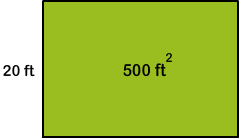12,585 Plays
Rate Quiz 0 stars
Quiz size:
Message preview:
Someone you know has shared Area of Squares and Rectangles: Word Problems quiz with you:

To play this quiz, click on the link below:

https://www.turtlediary.com/quiz/area-of-squares-rectangles-word-problems.html

Hope you have a good experience with this site and recommend to your friends too.

Login to rate activities and track progress.
Login to rate activities and track progress.

# Area of Squares and Rectangles: Word Problems

The area is the amount of surface enclosed by a closed figure.

Area of a rectangle = length × width

Area of a square = side × side

For example, consider this situation.

The area of a rectangular pool is 500 square feet. The width of the pool is 20 feet.

Let's find the length of the pool.We know,

 Length × width = area Length × 20 = 500 Length = 500 ÷ 20 = 25

So, the length of the pool is 25 feet.

ds

A B C D E F G H I J K L M N O P Q R S T U V W X Y Z